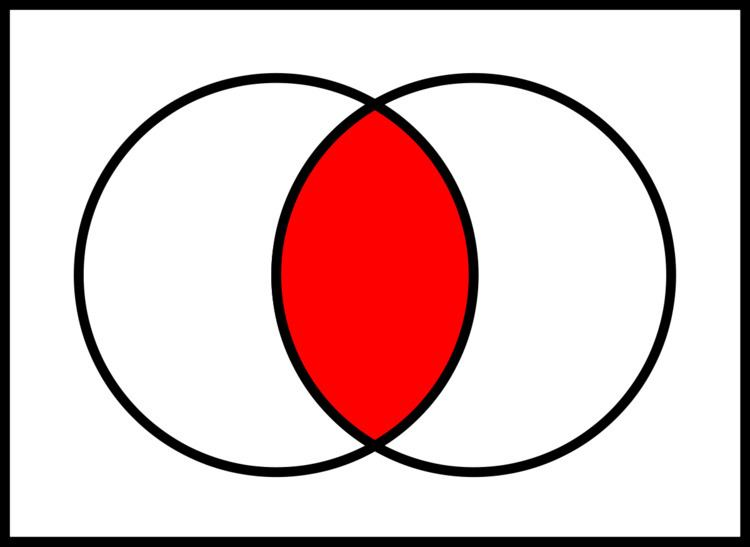# Logical conjunction

Updated onIn logic and mathematics, and is the truth-functional operator of logical conjunction; the and of a set of operands is true if and only if all of its operands are true. The logical connective that represents this operator is typically written as or .

## Contents

"A and B" is true only if A is true and B is true.

An operand of a conjunction is a conjunct.

Related concepts in other fields are:

• In natural language, the coordinating conjunction "and".
• In programming languages, the short-circuit and control structure.
• In set theory, intersection.
• In predicate logic, universal quantification.
• ## Notation

And is usually denoted by an infix operator: in mathematics and logic, or × ; in electronics, ; and in programming languages, `&`, `&&`, or `and`. In Jan Łukasiewicz's prefix notation for logic, the operator is K, for Polish koniunkcja.

## Definition

Logical conjunction is an operation on two logical values, typically the values of two propositions, that produces a value of true if and only if both of its operands are true.

The conjunctive identity is 1, which is to say that AND-ing an expression with 1 will never change the value of the expression. In keeping with the concept of vacuous truth, when conjunction is defined as an operator or function of arbitrary arity, the empty conjunction (AND-ing over an empty set of operands) is often defined as having the result 1.

## Truth table

The truth table of   A B :

## Introduction and elimination rules

As a rule of inference, conjunction introduction is a classically valid, simple argument form. The argument form has two premises, A and B. Intuitively, it permits the inference of their conjunction.

A, B. Therefore, A and B.

or in logical operator notation:

A , B A B

Here is an example of an argument that fits the form conjunction introduction:

Bob likes apples. Bob likes oranges. Therefore, Bob likes apples and oranges.

Conjunction elimination is another classically valid, simple argument form. Intuitively, it permits the inference from any conjunction of either element of that conjunction.

A and B. Therefore, A.

...or alternately,

A and B. Therefore, B.

In logical operator notation:

A B A

...or alternately,

A B B

## Properties

commutativity: yes

associativity: yes

distributivity: with various operations, especially with or

idempotency: yes

monotonicity: yes

truth-preserving: yes
When all inputs are true, the output is true.

falsehood-preserving: yes
When all inputs are false, the output is false.

Walsh spectrum: (1,-1,-1,1)

Nonlinearity: 1 (the function is bent)

If using binary values for true (1) and false (0), then logical conjunction works exactly like normal arithmetic multiplication.

## Applications in computer engineering

In high-level computer programming and digital electronics, logical conjunction is commonly represented by an infix operator, usually as a keyword such as "`AND`", an algebraic multiplication, or the ampersand symbol "`&`". Many languages also provide short-circuit control structures corresponding to logical conjunction.

Logical conjunction is often used for bitwise operations, where `0` corresponds to false and `1` to true:

• `0 AND 0`  =  `0`,
• `0 AND 1`  =  `0`,
• `1 AND 0`  =  `0`,
• `1 AND 1`  =  `1`.
• The operation can also be applied to two binary words viewed as bitstrings of equal length, by taking the bitwise AND of each pair of bits at corresponding positions. For example:

• `11000110 AND 10100011`  =  `10000010`.
• This can be used to select part of a bitstring using a bit mask. For example, `10011101 AND 00001000`  =  `00001000` extracts the fifth bit of an 8-bit bitstring.

In computer networking, bit masks are used to derive the network address of a subnet within an existing network from a given IP address, by ANDing the IP address and the subnet mask.

Logical conjunction "`AND`" is also used in SQL operations to form database queries.

The Curry–Howard correspondence relates logical conjunction to product types.

## Set-theoretic correspondence

The membership of an element of an intersection set in set theory is defined in terms of a logical conjunction: xAB if and only if (xA) ∧ (xB). Through this correspondence, set-theoretic intersection shares several properties with logical conjunction, such as associativity, commutativity, and idempotence.

## Natural language

As with other notions formalized in mathematical logic, the logical conjunction and is related to, but not the same as, the grammatical conjunction and in natural languages.

English "and" has properties not captured by logical conjunction. For example, "and" sometimes implies order. For example, "They got married and had a child" in common discourse means that the marriage came before the child. The word "and" can also imply a partition of a thing into parts, as "The American flag is red, white, and blue." Here it is not meant that the flag is at once red, white, and blue, but rather that it has a part of each color.

Topics